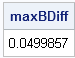In statistical programming, I often test a program by running it on a problem for which I know the correct answer. I often use a single expression to compute the maximum value of the absolute difference between the vectors:

`maxDiff = max( abs( z-correct ) ); /* largest absolute difference */`

In this expression, z is the vector that I have computed and correct is the correct answer.

Let's break this expression down into pieces:

• (z - correct) is the difference between the two vectors. They must have the same number of elements in order for this expression to make sense. The result is the elementwise difference. That is, the expression resolves to a temporary vector (let's call it diff) that is the same length as z and the ith element is equal to z[i]- correct[i].
• The ABS function returns a vector that contains the absolute values of each element of its argument.
• The MAX function returns a scalar value that is the maximum value of the elements of its argument.
Consequently, the expression returns a scalar value that is the largest absolute difference between the vector z and the vector correct.

For example, last week I showed how you can use the DIF function to compute simple finite-difference approximations to derivatives. In that article, I computed an approximate derivative to the sine function and compared it to the true derivative, as follows:

```proc iml; h = 0.1; x = T( do(0, 6.28, h) ); /* x in [0, 2 pi] */ y = sin(x); approx = dif(y, 1) / h; /* f'(x) ~ (f(x)-f(x-h))/h */ correct = cos(x); /* true derivatives at x */ maxBDiff = max(abs(approx - correct)); /* find maximum difference */ print maxBDiff;```The output tells me that the approximate derivative and the true value differ by about 0.05 for some value of the x vector.

It is interesting to note that you can use the exact same expression if correct is a scalar value. In this case, you are computing the maximum absolute deviation between a vector of values and a target value.

### Other ways to compute differences and deviations

In statistics, a difference between two values is called a deviation, especially when one expression is an estimate and another is an expected value. In the language of statistics, the expression in the previous section is similar to the maximum absolute deviation. There are other statistical concepts that you can use to measure the difference between two vectors, or between a vector and a target value:

• Mean absolute deviation: Instead of taking the maximum, you can compute the mean of the absolute differences: (abs(z-correct))[:]. Recall that the colon (:) subscript operator is a convenient way to compute the mean of a vector in the SAS/IML language.
• Median absolute deviation: The mean absolute deviation is sensitive to large values. A robust alternative is the median absolute deviation (MAD), which you can compute by using the built-in MAD function: mad(z-correct)
• Sum of squared errors: You can use the SSQ function to compute the sum of squared errors between two vectors: ssq(z-correct).
• Mean squared error: Compute the mean of the squared deviations. Define diff = z-correct. Then ssq(diff)/countn(diff) is the mean squared error. I use the COUNTN function rather than the NCOL function in case there are missing values in the diff vector.
• Root mean squared error: The square root of the previous quantity is the root mean squared error.

There are other measures that you can use (such as relative quantities), but these are some common ways to compute a measure of how much one vector of values differs from another.

Share